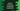# Python dictionary setdefault() method explanation with example## Python dictionary setdefault() method:

Python dictionary setdefault method is used to set a value for a key in dictionary if the key is not available. If the key is available, it returns the value for that key.

In this post, we will learn how to use setdefault with examples.

### syntax and return value:

The syntax of setdefault is as below:

``dic.setdefault(k[, v])``

Here, k is the key and v is the value we want to set for this key. The value is optional. If we don’t assign anything for v, it will take None by default.

The return value of this method is the value v if key k is not in the given dictionary dic. If the key k is in the dictionary dic, it returns the value for that key.

Let’s try it with different examples.

### setdefault example when key is in the dictionary:

Let’s take a look at the below example:

``````given_dict = {'one': 1, 'two': 2}

print(given_dict.setdefault('two', 4))

print(given_dict)``````

Here,

• given_dict is the dictionary given.
• The second line is using setdefault to set the value 4 for the key two.
• The last line is printing the dictionary.

If you run this program, it will print the below output:

``````2
{'one': 1, 'two': 2}``````

We are using setdefault with an existing key. So, it is not changing the value for that key. Also the existing value, i.e. 2 is returned.

### setdefault example when key is not in the dictionary:

Now, let’s change the above example as like below:

``````given_dict = {'one': 1, 'two': 2}

print(given_dict.setdefault('three', 3))

print(given_dict)``````

Now,

• we are assigning a value for three.
• Since three is not in the dictionary, it will add this key and value to the dictionary and setdefault will return 3.

It will print the below output:

``````3
{'one': 1, 'two': 2, 'three': 3}``````